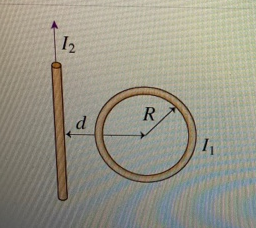# Problem: The wire carries current I2, which is 20 times the current I1 of the loop; the direction of the current in the wire is shown. The magnetic field in the center of the loop is zero. The radius R of the loop is 1.5 cm.A.) What is the direction of the current in the loop?B.) What is the distance d from the wire to the center of the loop?

###### FREE Expert Solution

In this problem, we have a combination of the magnetic field due to straight currents and loops.

Magnetic field around a wire carrying current:

$\overline{){\mathbf{B}}{\mathbf{=}}\frac{{\mathbf{\mu }}_{\mathbf{0}}\mathbf{i}}{\mathbf{2}\mathbf{\pi }\mathbf{r}}}$ r is the distance from the wire to a point perpendicular to the wire.

The magnetic field produced by a current loop:

$\overline{){\mathbf{B}}{\mathbf{=}}{\mathbf{N}}\frac{{\mathbf{\mu }}_{\mathbf{0}}\mathbf{i}}{\mathbf{2}\mathbf{r}}}$ r is the radius of the loop

96% (378 ratings)###### Problem Details

The wire carries current I2, which is 20 times the current I1 of the loop; the direction of the current in the wire is shown. The magnetic field in the center of the loop is zero. The radius R of the loop is 1.5 cm.

A.) What is the direction of the current in the loop?

B.) What is the distance d from the wire to the center of the loop?